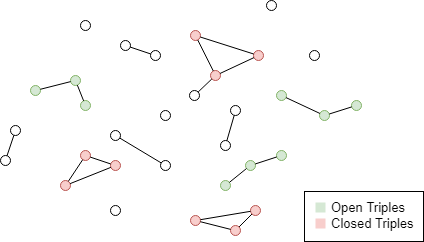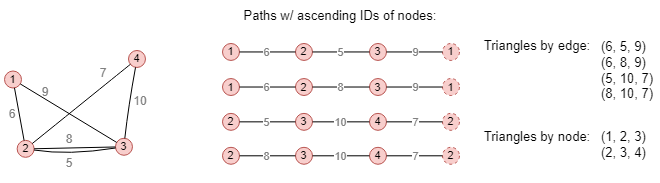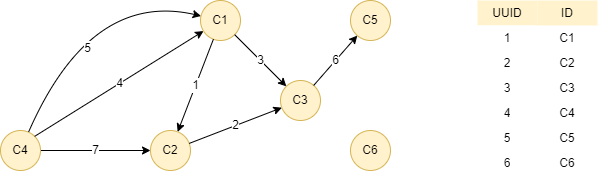# Change Nickname

Current Nickname:

Certifications

Certificate Issued at Valid until Serial No. File
Serial No. Valid until

Not having one? Apply now! >>>

Invoice

ProductName CreateTime ID Price File
ProductName CreateTime ID Price File

# Triangle Counting

## Overview

Triangle Counting algorithm is used to count the number of triangles made up of nodes and edges in an undirected graph, and return the nodes or edges of each triangle. Triangles reflect the ability to form loops between any three nodes in the graph, it is one of the basic concepts when calculating the global aggregation coefficient.

Triangle Counting is a general purpose graph algorithm which is commonly used in social network discovery, community identification, compactness analysis, stability analysis, etc. For example, the number of triangles formed by transactions between accounts issued by a financial institution shows the degree of connectivity and connection tightness of the accounts under this financial institution.

## Basic Concept

### Triplet

Triplet, or Triples, is one of the basics in topology that refers to the 3 nodes in a simple graph connected by 2~3 edges (there is at most one edge between any two nodes in a simple graph). Among them, triplet connected by two edges is called Open Triplet, and triplet connected by three edges is called Closed Triplet.When calculating a simple graph, the global aggregation coefficient can be obtained by dividing the number of closed triplets by the number of all triplets. The global aggregation coefficient reflects the degree of interconnection between the adjacent nodes of one node in the graph from the perspective of triplets.

### Triangle

Triangle that Triangle Counting algorithm calculates is closed triplet. The definition of closed triplet is given in simple graph; for complex graph, multiple edges may exist between any two nodes, thus the definition of triangle is divided into two categories:

• Triangles assembled by edge
• Triangles assembled by node

The process of assembling triangles by edge can be understood as looking for loops in the graph that connect any three nodes. Repeated results can be produced by different start node and direction of the path since it is loop, but we can sort the ID of the nodes in the path in some order (such as the simple ascending, descending order and so on) to deduplicate:A total of 4 loops are found in the graph above, i.e. 4 triangles assembled by edge. If ignores the edges in these 4 loops, but view the loops as a sequence of nodes, there are 2 triangles assembled by node after removing the deduplicated results. The process of assembling triangles by node is equivalent to merging multiple edges between any two nodes into one edge (that is, compressing the complex graph into a simple graph), and then looking for loops that connect any three nodes. In complex graph, the number of triangles assembled by edge is usually greater than the number of triangles assembled by node.

The assembling principle to count triangles depends on the application scenario. In general, application scenarios dominated by social network analysis adopt the assembling by node principle, while financial network analysis prefers assembling by edge principle with the purpose to show how tight nodes are connected.

## Special Case

### Lonely Node, Disconnected Graph

Lonely node does not form triangles.

Disconnected graph assembles triangles in its connected components.

### Self-loop Edge

Self-loop edge is not one of the elements to form triangles, it does not participate in the assembling of triangles either.

### Directed Edge

For directed edges, Triangle Counting algorithm ignores the direction of edges but calculates them as undirected edges.

## Results and Statistics

Take the graph below as an example, run the Triangle Counting algorithm in the graph:Algorithm results 1: Calculate triangles assembled by edge, return `triangle_count` or `edge1`, `edge2`, `edge3` according to the result type

triangle_count
3
edge1 edge2 edge3
4 1 7
5 1 7
3 1 2

Algorithm statistics 1: Number of triangles `triangle_count`

triangle_count
3

Algorithm results 2: Calculate triangles assembled by node, return `triangle_count` or `node1`, `node2`, `node3` according to the result type

triangle_count
2
node1 node2 node3
4 1 2
3 1 2

Algorithm statistics 2: Number of triangles `triangle_count`

triangle_count
2

## Command and Configuration

• Command: `algo(triangle_counting)`
• Configurations for the parameter `params()`:
Name
Type
Default
Specification
Description
type int 1 1 or 2 1 or if not set means to count triangles by edge, 2 means to count triangles by node
result_type int 1 1 or 2 1 or if not set means to return the number of triangles, 2 means to return the nodes or edges that form the triangle
limit int -1 >=-1 Number of results to return; return all results if sets to -1 or not set

Example: Calculate triangles by edge in the whole graph, return the number of triangles

``````algo(triangle_counting).params({type: 1}) as tri
return tri
``````

Example: Calculate triangles by node in the whole graph, return 1 group of nodes that form the triangle

``````algo(triangle_counting).params({
type: 2,
result_type: 2,
limit: 1}) as tri
return tri
``````

## Algorithm Execution

#### 1. File Writeback

Configuration
Data in Each Row
filename `triangle_count` or `edge1`, `edge2`, `edge3` or `node1`, `node2`, `node3`

Example: Calculate triangles by node in the whole graph, write the algorithm results back to file named test

``````algo(triangle_counting).params({
type: 2,
result_type: 2
}).write({
file:{
filename: "test"
}})
``````

#### 2. Property Writeback

Not supported by this algorithm.

#### 3. Statistics Writeback

Name Data Type Description
`triangle_count` int Number of triangles

Example: Example: Calculate triangles by edge in the whole graph, write the algorithm statistics back to task information

``````algo(triangle_counting).params({
result_type: 1
}).write()
``````

### Direct Return

Alias Ordinal Type
Description
Column Name
0 KV or []perTriangle Nodes or edges that form the triangle or Number of triangles `triangle_count` or `edge1`, `edge2`, `edge3` / `node1`, `node2`, `node3`

Example: Calculate triangles by edge in the whole graph, define algorithm results (number of triangles) as alias named count, return the results

``````algo(triangle_counting).params({
result_type: 1
}) as count
return count
``````

Example: Calculate triangles by edge in the whole graph, define algorithm results (edges of triangles) as alias named triangles, return the results

``````algo(triangle_counting).params({
result_type: 2
}) as triangles
return triangles
``````

### Streaming Return

Alias Ordinal Type
Description
Column Name
0 KV or []perTriangle Nodes or edges that form the triangle or Number of triangles `triangle_count` or `edge1`, `edge2`, `edge3` / `node1`, `node2`, `node3`

Example: Calculate triangles by node in the whole graph, return all information of nodes that form the triangles

``````algo(triangle_counting).params({
type: 2,
result_type:2
}).stream() as tri
find().nodes({_uuid == tri.node1 || _uuid == tri.node2 || _uuid == tri.node3}) as nodes
return nodes{*}
``````

### Real-time Statistics

Alias Ordinal Type
Description
Column Name
0 KV Number of triangles `triangle_count`

Example: Calculate triangles by edge in the whole graph, define algorithm statistics as alias named sta, return the statistics

``````algo(triangle_counting).params({
result_type: 1
}).stats() as sta
return sta
``````